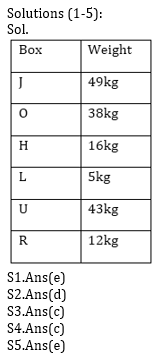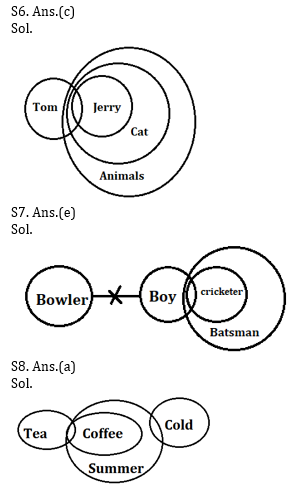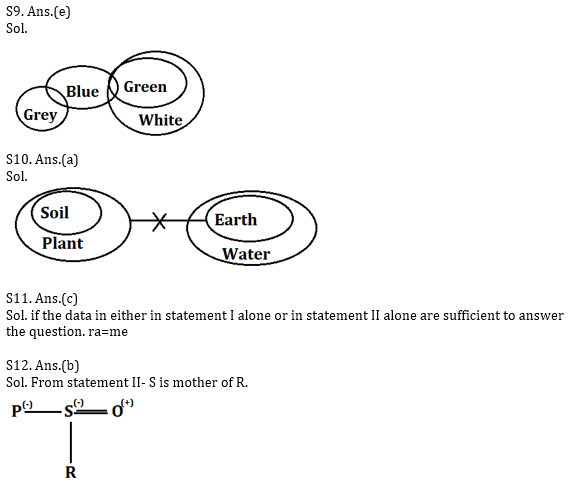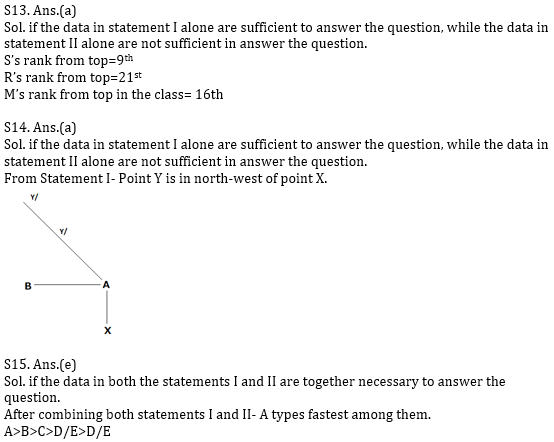Latest Banking jobs   »   Reasoning Ability Quiz for Prelims Exams-...

# Reasoning Ability Quiz for Prelims Exams- SBI & IBPS 2020- 3rd December

Directions (1-5): Study the information carefully and answer the questions given below.
Six boxes H, J, L, O, R and U are placed one above another in a stack. Each box has different weight i.e. 16kg, 5kg, 43kg, 49kg, 12kg and 38kg. All information is not necessarily in same order. Box L has weight that is prime number. One box is placed between L and O and O’s weight is 38kg. Box O placed above L. The box whose weight is perfect square is placed just above Box O. Two boxes are place between box H and box R which is not placed above O. Box R isn’t placed just above or below box L. One box is placed between the box which weight is 16 and the box which weight is 49. Box R weight isn’t a perfect square. One box is placed between the box which weight is 16 and the box which weight is 43. Box J is placed above the box U, which isn’t placed above box H. Box H weight is not more than 38kg.

Q1. Which of the following box weight is 49kg?
(a) H
(b) O
(c) R
(d) U
(e) None of these

Q2. How many boxes are placed above box U?
(a) One
(b) Three
(c) None
(d) Four
(e) five

Q3. Which of the following box weight is 5kg?
(a) O
(b) H
(c) L
(d) R
(e) None of these

Q4. How many boxes have weight more than box H?
(a) Two
(b) One
(c) Three
(d) Four
(e) None of these

Q5. What is the difference between the weight of box U and the box which is just above box H?
(a) 26
(b) 7
(c) 22
(d) 4
(e) None of these

Directions (6-10): In each of the questions below are given some statements followed by some Conclusions. You have to take the given statements to be true even, if they seem to be at variance from commonly known facts. Read all the conclusions and then decide which of the given conclusions logically follows from the given statements disregarding commonly known facts.

Q6.
Statements:
Some Tom are Jerry
All Jerry are Cat
All cat are Animal
Conclusions:
I: Some Tom are not Animals
II: All Tom are animals

(a) If only conclusion I follows.
(b) If only conclusion II follows.
(c) If either conclusion I or II follows.
(d) If neither conclusion I nor II follows.
(e) If both conclusions I and II follow.

Q7.
Statements:
Some Boy are Cricketer
All Cricketer are Batsman
No Boy is Bowler
Conclusions:
I: Some Cricketer is not bowler
II: All Batsman can never be bowler

(a) If only conclusion I follows.
(b) If only conclusion II follows.
(c) If either conclusion I or II follows.
(d) If neither conclusion I nor II follows.
(e) If both conclusions I and II follow.

Q8.
Statements:
All coffee are summer
Some tea is coffee
Some summer are cold
Conclusions:
I. All tea being cold is a possibility
II. Some coffee are definitely not cold.

(a) If only conclusion I follows.
(b) If only conclusion II follows.
(c) If either conclusion I or II follows.
(d) If neither conclusion I nor II follows.
(e) If both conclusions I and II follow.

Q9.
Statements:
Some blue are green
Some grey is blue
All green are white
Conclusions:
I. Some grey is white is a possibility
II. All green are grey is a possibility

(a) If only conclusion I follows.
(b) If only conclusion II follows.
(c) If either conclusion I or II follows.
(d) If neither conclusion I nor II follows.
(e) If both conclusions I and II follow.

Q10.
Statements:
All soil are plant
No earth is plant
All earth are water
Conclusions:
I. Some plant which are water are also soil.
II. No water is soil

(a) If only conclusion I follows.
(b) If only conclusion II follows.
(c) If either conclusion I or II follows.
(d) If neither conclusion I nor II follows.
(e) If both conclusions I and II follow.

Directions (11-15): Each of the following questions below consists of a question and two statements numbered I and II given below it. You have to decide whether the data provided in the statements are sufficient to answer the question. Read both the statements and give answer.
(a) if the data in statement I alone are sufficient to answer the question, while the data in statement II alone are not sufficient in answer the question.
(b) if the data in statement II alone are sufficient to answer the question, while the data in statement I alone are not sufficient to answer the question.
(c) if the data in either in statement I alone or in statement II alone are sufficient to answer the question.
(d) if the data in both the statements I and II together are not sufficient to answer the question.
(e) if the data in both the statements I and II are together necessary to answer the question.

Q11. What does ‘ra’ mean in the code language?
I. ‘st qm ra’ means ‘Look at me’ and ‘qm ms st rt’ means ‘don’t look at him’ in that code language.
II. ‘ka tv ne ra’ means ‘take me and go’ and ‘vw wx si ra’ means ‘either me or you’ in that code language.

Q12. How is S related to R?
I. Q is the cousin of R and niece of S.
II. P is sister of S who is the wife of O who is the father of R.

Q13. What is M’s rank in the class of 40?
I. S, who is 9th from the top in the class is above R by 12 ranks who is below M by 5 ranks.
II. N, who is between M and Q, is 15th from the bottom.

Q14. Point Y is in which direction with respect to point X?
I. Point A is east of point B and north of point X. Point Y is north-west of point A.
II. Point X is east of point B and north of point A. Point Y is north of point A.

Q15. Who among A, B, C, D and E types fastest?
I. B types faster than E but he is not the fastest among them.
II. C types faster than D and E. C does not type as fast as A and B.

Practice More Questions of Reasoning for Competitive Exams:

##### Prelims Quiz Study Plan for SBI & IBPS Exams 2020

SolutionsPractice with Crash Course and Online Test Series for IBPS Clerk Prelims:×

Thank You, Your details have been submitted we will get back to you.Join India's largest learning destination

What You Will get ?

•Job Alerts
•Daily Quizzes
•Subject-Wise Quizzes
•Current Affairs
•Previous year question papers
•Doubt Solving session

ORJoin India's largest learning destination

What You Will get ?

•Job Alerts
•Daily Quizzes
•Subject-Wise Quizzes
•Current Affairs
•Previous year question papers
•Doubt Solving session

ORJoin India's largest learning destination

What You Will get ?

•Job Alerts
•Daily Quizzes
•Subject-Wise Quizzes
•Current Affairs
•Previous year question papers
•Doubt Solving session

Enter the email address associated with your account, and we'll email you an OTP to verify it's you.Join India's largest learning destination

What You Will get ?

•Job Alerts
•Daily Quizzes
•Subject-Wise Quizzes
•Current Affairs
•Previous year question papers
•Doubt Solving session

Enter OTP

Please enter the OTP sent to
/6

Did not recive OTP?

Resend in 60sJoin India's largest learning destination

What You Will get ?

•Job Alerts
•Daily Quizzes
•Subject-Wise Quizzes
•Current Affairs
•Previous year question papers
•Doubt Solving sessionJoin India's largest learning destination

What You Will get ?

•Job Alerts
•Daily Quizzes
•Subject-Wise Quizzes
•Current Affairs
•Previous year question papers
•Doubt Solving session

Almost there

+91Join India's largest learning destination

What You Will get ?

•Job Alerts
•Daily Quizzes
•Subject-Wise Quizzes
•Current Affairs
•Previous year question papers
•Doubt Solving session

Enter OTP

Please enter the OTP sent to Edit Number

Did not recive OTP?

Resend 60

By skipping this step you will not recieve any free content avalaible on adda247, also you will miss onto notification and job alerts

Are you sure you want to skip this step?

By skipping this step you will not recieve any free content avalaible on adda247, also you will miss onto notification and job alerts

Are you sure you want to skip this step?